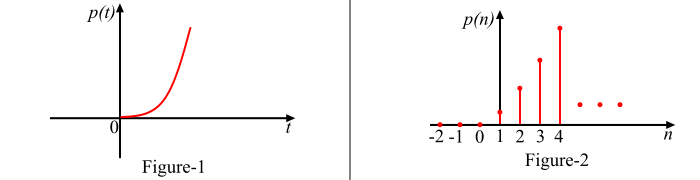# What is a Unit Parabolic Signal?

When a signal gives the constant acceleration distinction of actual input signal, such a signal is known as parabolic signal or parabolic function. It is also known as unit acceleration signal. The unit parabolic signal starts at t = 0.

## Continuous-Time Unit Parabolic Signal

The continuous-time unit parabolic signal is a unit parabolic signal which is defined for every instant of positive time. It is denoted by p(t). Mathematically, p(t) is given as,

$$\mathrm{p(t)=\left\{\begin{matrix} \frac{t^{2}}{2}\; for\: t\geq 0\ 0\; for\; t< 0\ \end{matrix}\right.}$$

Also,

$$\mathrm{p(t)=\frac{t^{2}}{2}\;u(t)}$$

The graphical representation of the continuous-time parabolic signal p(t) is shown in Figure-1.## Discrete-Time Unit Parabolic Signal

The discrete-time unit parabolic sequence is a unit parabolic signal which is defined only at discrete instants of positive time n. It is denoted by p(n). Mathematically, p(n) is given as,

$$\mathrm{p(n)=\left\{\begin{matrix} \frac{n^{2}}{2}\; for\: n\geq 0\ 0\; for\; n< 0\ \end{matrix}\right.}$$

$$\mathrm{p(n)=\frac{n^{2}}{2}\;u(n)}$$

The graphical representation of discrete-time unit parabolic signal p(n) is shown in Figure-2.

## Relationship between Unit Parabolic Signal and Unit Ramp Signal

The unit parabolic signal can be obtained by integrating the unit ramp signal with respect to time. In other words, the unit ramp function is the time derivative of the unit parabolic function, i.e.,

$$\mathrm{p(t)=\int r(t)dt=\int t\: dt=\frac{t^{2}}{2}\; \; for\: t\geq 0}$$

Or

$$\mathrm{r\left ( t \right )=\frac{\mathrm{d} }{\mathrm{d} t}p(t)}$$

## Relationship between Unit Parabolic Signal and Unit Step Signal

The unit parabolic signal can be obtained by double integrating the unit step signal. In other words, the unit step signal is a double derivative of parabolic signal, i.e.,

$$\mathrm{p(t)=\iint\: u(t)dt\: dt=\iint\: 1\: dt\: dt=\int t\: dt=\frac{t^{2}}{2}\; \; for\: t\geq 0 }$$

or

$$\mathrm{u(t)=\frac{\mathrm{d} ^{2}}{\mathrm{d} t^{2}}p(t)}$$